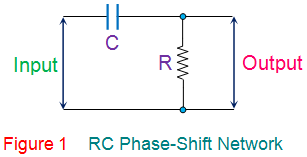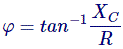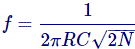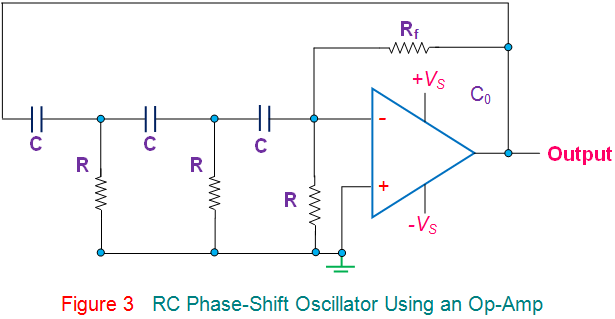# RC Phase Shift Oscillator

RC phase-shift oscillators use resistor-capacitor (RC) network (Figure 1) to provide the phase-shift required by the feedback signal. They have excellent frequency stability and can yield a pure sine wave for a wide range of loads.Ideally a simple RC network is expected to have an output which leads the input by 90o.

However, in reality, the phase-difference will be less than this as the capacitor used in the circuit cannot be ideal. Mathematically the phase angle of the RC network is expressed asWhere, XC = 1/(2πfC) is the reactance of the capacitor C and R is the resistor. In oscillators, these kind of RC phase-shift networks, each offering a definite phase-shift can be cascaded so as to satisfy the phase-shift condition led by the Barkhausen Criterion.

One such example is the case in which RC phase-shift oscillator is formed by cascading three RC phase-shift networks, each offering a phase-shift of 60o, as shown by Figure 2.Here the collector resistor RC limits the collector current of the transistor, resistors R1 and R (nearest to the transistor) form the voltage divider network while the emitter resistor RE improves the stability. Next, the capacitors CE and Co are the emitter by-pass capacitor and the output DC decoupling capacitor, respectively. Further, the circuit also shows three RC networks employed in the feedback path.

This arrangement causes the output waveform to shift by 180o during its course of travel from output terminal to the base of the transistor. Next, this signal will be shifted again by 180o by the transistor in the circuit due to the fact that the phase-difference between the input and the output will be 180o in the case of common emitter configuration. This makes the net phase-difference to be 360o, satisfying the phase-difference condition.
One more way of satisfying the phase-difference condition is to use four RC networks, each offering a phase-shift of 45o. Hence it can be concluded that the RC phase-shift oscillators can be designed in many ways as the number of RC networks in them is not fixed. However it is to be noted that, although an increase in the number of stages increases the frequency stability of the circuit, it also adversely affects the output frequency of the oscillator due to the loading effect.
The generalized expression for the frequency of oscillations produced by a RC phase-shift oscillator is given byWhere, N is the number of RC stages formed by the resistors R and the capacitors C.
Further, as is the case for most type of oscillators, even the RC phase-shift oscillators can be designed using an OpAmp as its part of the amplifier section (Figure 3). Nevertheless, the mode of working remains the same while it is to be noted that, here, the required phase-shift of 360o is offered collectively by the RC phase-shift networks and the Op-Amp working in inverted configuration.Further, it is to be noted that the frequency of the RC phase-shift oscillators can be varied by changing either the resistors or the capacitors. However, in general, the resistors are kept constant while the capacitors are gang-tuned. Next, by comparing the RC phase-shift oscillators with LC oscillators, one can note that, the former uses more number of circuit components than the latter one. Thus, the output frequency produced from the RC oscillators can deviate much from the calculated value rather than in the case of LC oscillators. Nevertheless, they are used as local oscillators for synchronous receivers, musical instruments and as low and/or audio-frequency generators.

Want To Learn Faster? 🎓
Get electrical articles delivered to your inbox every week.
No credit card required—it’s 100% free.